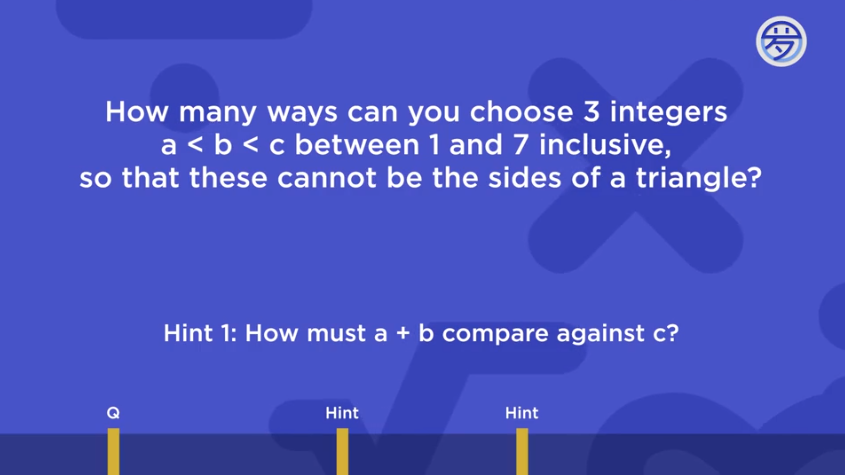# How I did it

• Follow my train of thought here:

I forgot how to use grid method and I didn't see how it would work, so I listed it all out by hand.

a+b has to be less than or equal to c to make this work, so (the addition means a+b)

1 Nothing
2 1+1
3 1+2 1+1
4 2+2 1+2 1+1
5 2+3 2+2 1+2 1+1
6 3+3 2+3 2+2 1+2 1+1
7 3+4 3+3 2+3 2+2 1+2 1+1

Notice how it all stacks up? Problem is, my answer was 21 with this method, and there was no choice of 21. I didn't know what else to do, so I picked (spoiler alert!!!)

22

And I got it right lol. Was there something wrong with my method though?

• Note: some of them may be in the wrong order but you can just flip it around

• Another note: I thought about it over and a lot of it doesn't make sense. Still if you can correct this strategy so it works thanks in advance.

• @The-Darkin-Blade Hi there! I'll start by giving the original Your Turn question again just to make it easier to follow along: We want $$3$$ integers $$a < b < c$$ between $$1$$ and $$7$$ inclusive (which means that we are allowed to set them equal to $$1$$ or $$7,$$ such that these $$a, b,$$ and $$c$$ cannot be the sides of a triangle.Note that none of the $$a, b$$ or $$c$$ are allowed to be equal to each other, so that means that some of the ways in your chart above aren't valid. For example, these aren't valid:

\begin{aligned} c &= 2; 1 + 1 \\ c &= 3; 1 + 1 \\ c &=4; 2 + 2 \text{ and } 1 + 1 \\ \dots \end{aligned}

So all of the ways like $$1 + 1, 2 + 2, 3 + 3 \ldots$$ aren't possible, since here $$a = b.$$

Another thing I noticed is that you seem to be carrying over the previous line to the next, while tacking on one more sum. Like, for the line $$c = 3,$$ you copy the $$1 + 2; 1 + 1$$ over to the next line, while adding a $$2 + 2$$ since the new sum can be $$4.$$ However, notice that in the next line, we actually should add to the ways which have the form $$1 + \text{ anything }.$$ For example, the line $$c = 4$$ should also include $$1 + 3,$$ even though $$1 + 3$$ doesn't occur in the previous line where $$c = 3.$$

So, a complete list of all the ways would look more like this:

\begin{aligned} c &=1 \implies \text{ Nothing } \\ c &= 2 \implies \text{ Nothing } \\ c &= 3 \implies 1 + 2 \\ c &= 4 \implies 1 + 2; \text{ } 1 + 3 \\ c &= 5 \implies 1 + 2; \text{ } 1 + 3; \text{ } \textcolor{red}{1 + 4}; \text{ } 2 + 3 \\ c &= 6 \implies 1 + 2; \text{ } 1 + 3; \text{ } 1 + 4; \text{ } \textcolor{red}{1 + 5}; \text{ } 2 + 3; \text{ } 2 + 4 \\ c &= 7 \implies 1 + 2; \text{ } 1 + 3; \text{ } 1 + 4; \text{ } 1 + 5; \textcolor{red}{1 + 6}; \text{ } 2 + 3; \text{ } 2 + 4; \text{ } \textcolor{red}{2 + 5}; \text{ } 3 + 4 \\ \end{aligned}

I've highlighted some of these entries in $$\textcolor{red}{\text{red}}$$ since they are additions to the pattern that we borrow from the previous line.

Now, if we add up all of these ways, we have $$1$$ on the third row, $$2$$ on the fourth row, $$4$$ on the fifth row, $$6$$ on the sixth row, and $$9$$ on the seventh row, which adds up to $$1 + 2 + 4 + 6 + 9 = \boxed{22}.$$

I hope this helps! Let me know if anything I said was confusing. Good luck with the rest of the course; you're almost done!

• @The-Rogue-Blade Your idea is correct but you missed some pairs and also, a<b so pairs with the same numbers are incorrect. When prof. loh drew a line across the chart he did it above the middle, so he excluded all the pairs with the same numbers.
These shouldn't be included2: 1,1
3: 1,1
4: 2,2 1,1
5: 2,2 1,1
6: 3,3 2,2 1,1
7: 3,3 2,2 1,1

I really liked the way you did the question and it would be quite useful if I didn't want to draw a graph